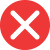4th

Correct spelling, explanation: 4th is the correct form because of how we create ordinal numbers. We do so by adding the -th suffix to the cardinal number – for example six – sixth; hence four – fourth. We add -th in numeral form as well: 6 – 6th, 4 – 4th. There are three other suffixes used for ordinal numbers: st, nd, and rd. They are irregular and used only for 1, 2, and 3. Therefore rd can be used only with the number 3 or numbers ending in three (for example 3rd, 33rd, 173rd, etc). Therefore 4th is the correct form and 4rd is a mistake.

Definition of 4th:
numeral, the ordinal number between 3rd and 5th
He was 4th in the race! That’s too bad he didn’t make it onto the podium.
This is the 4th time I’ve told you to take out the trash!4rd

Incorrect spelling, explanation: 4rd is the wrong form because, with most numbers, including the number 4, we use -th as the suffix for ordinal numbers (9th, 4th, 66th, etc.), not -rd. -rd suffix is used only with number 3, for example, 43rd, 3rd, or 953rd. -th is a regular suffix used with most numbers, so the correct form is 4th.4st

Incorrect spelling4nd

Incorrect spelling